#### Vba Code to Sort Data

In this article, we are going to learn how to sort data in columns with VBA instead of using Excel sorting. You will learn how to do this by doing an example. We are going to random sort the data in columns using VBA.

## Random numbers

To do this follow these steps:

First write any 5 random names in column A. Now you are going to write 5 random numbers to column B.

## The ribbon

Now place a command button on your Excel sheet. To open the developer tab right-click anywhere on the ribbon (the top menu) and select “Customize the Ribbon” from the drop down menu.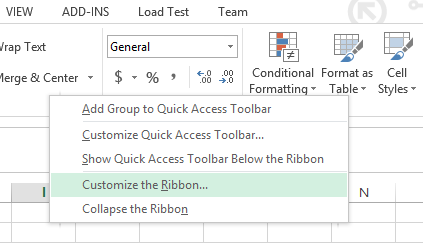Click on the DEVELOPER check box. It is under “Customize the Ribbon Main Tabs”.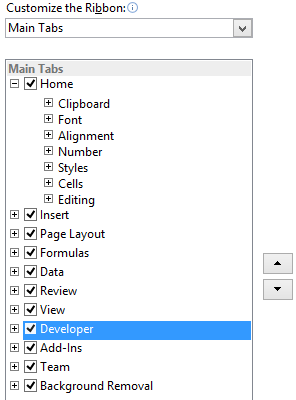The developer tap is now visible and is present next to the view tab on the top menu bar. Click on the DEVELOPER tab and select “Insert”.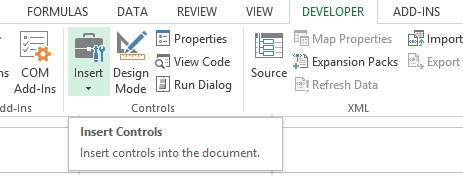## The button

Select the Command button in the ActiveX Controls.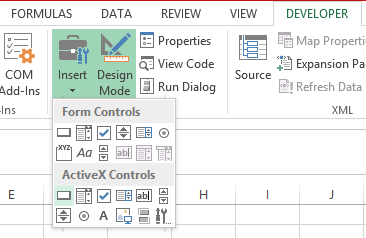Drag the command button on your Excel worksheet. Right-click on the command button (make sure Design Mode is selected) and go to properties. Write Rand Sort Names in the “Caption” field.

## The code

A new window (Visual Basic Editor) will open which will have a dialog box in the center. You will write the code in this dialog box.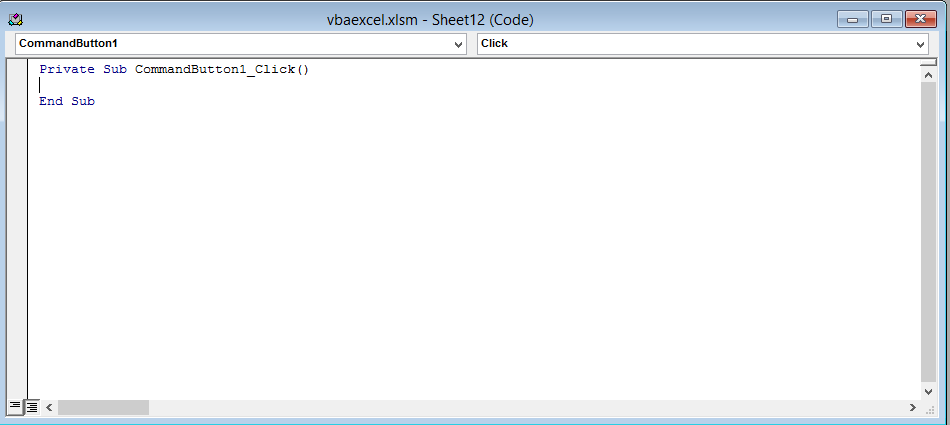### Variables

Now declare four variables.

```Dim tempString As String, tempInteger As Integer, a As Integer,
b As Integer```

After this, use the worksheet function RandBetween.

```For a = 1 To 5
Cells (a, 2).Value = WorksheetFunction.RandBetween(0, 1000)
Next a```

### Double loop

You will use the numbers next to each name to sort the names. The name with the lowest number first, the name with the second lowest number, second, etc.

Now use a Double Loop.

```For a = 1 To 5
For b = a + 1 To 5```

Add the following line of code:

`If Cells (b, 2).Value < Cells (a, 2).Value Then`

Example: for a = 1 and b = 2, Sarah and Laura are compared. Because Sarah has a lower number, you swap Sarah and Laura. Sarah is at the first position now.

If the condition is true, swap the names.

```tempString = Cells (a, 1).Value
Cells (a, 1).Value = Cells (b, 1).Value
Cells (b, 1).Value = tempString```

And swap the numbers.

```tempInteger = Cells (a, 2).Value
Cells (a, 2).Value = Cells(b, 2).Value
Cells (b, 2).Value = tempInteger```

Close the IF statement

`End If`

Close both the FOR loops

```Next b
Next a```

### Code completed

This is how the complete code should look like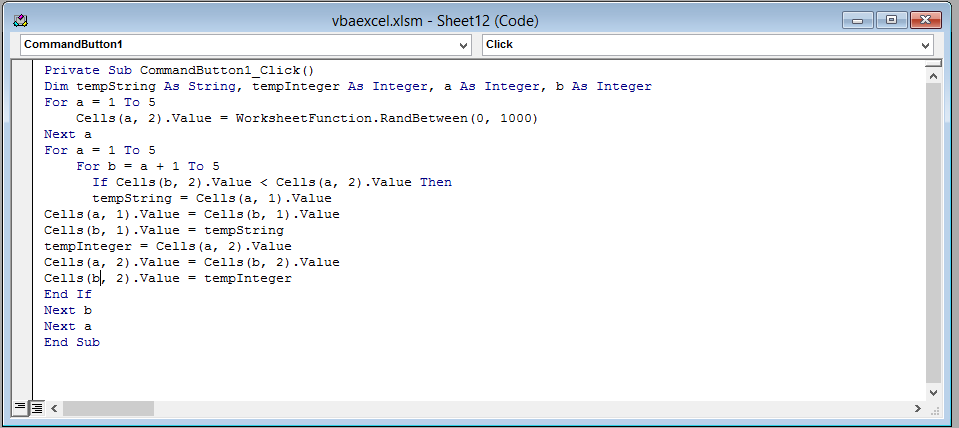After writing down the entire code close the window (Visual Basic Editor) by clicking on the cross(x) icon on the top right corner of the screen.

## The result

Click on the command button and see the result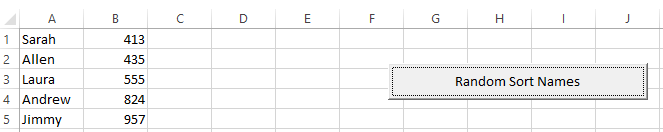You have now successfully sorted data and names using VBA.